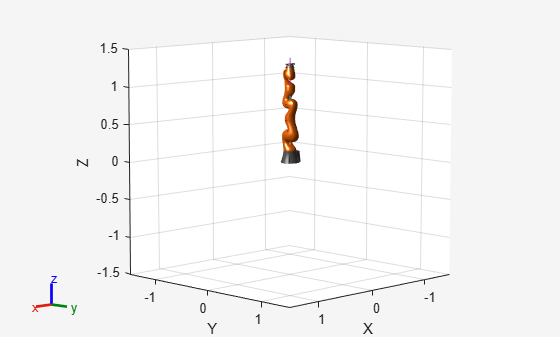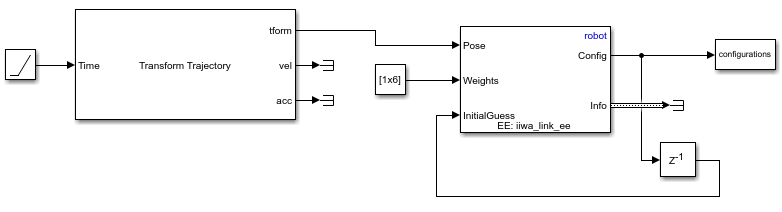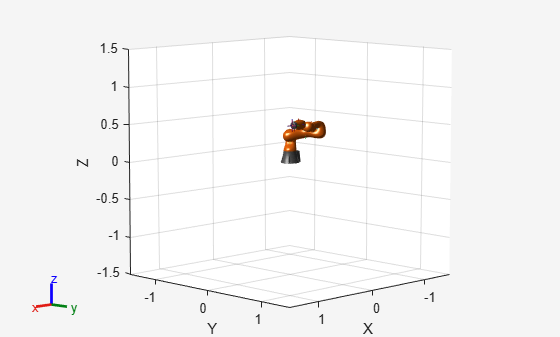# Execute Transformation Trajectory Using Manipulator and Inverse Kinematics

This example shows how to generate a transformation trajectory using the Transform Trajectory block and execute it for a manipulator robot using inverse kinematics.

Generate two homogenous transformations for the start and end points of the trajectory.

`tform1 = trvec2tform([0.25 -0.25 1])`
```tform1 = 4×4 1.0000 0 0 0.2500 0 1.0000 0 -0.2500 0 0 1.0000 1.0000 0 0 0 1.0000 ```
`tform2 = trvec2tform([0.25 0.25 0.5])`
```tform2 = 4×4 1.0000 0 0 0.2500 0 1.0000 0 0.2500 0 0 1.0000 0.5000 0 0 0 1.0000 ```

Import the robot model and specify the data format for Simulink®.

```robot = importrobot('iiwa14.urdf'); robot.DataFormat = 'column'; show(robot);```Open the model. The Transform Trajectory block interpolates between the initial and final transformation specified in the block mask. These transformations are fed to the Inverse Kinematics block to solve for the robot configuration that makes the end effector reach the desired transformation. The configurations are output to the workspace as `configurations`.

`open_system('transform_traj_ex1.slx')`Run the simulation and get the robot configurations.

`simOut = sim('transform_traj_ex1.slx')`
```simOut = Simulink.SimulationOutput: configurations: [7x1x52 double] tout: [52x1 double] SimulationMetadata: [1x1 Simulink.SimulationMetadata] ErrorMessage: [0x0 char] ```

Show the robot configurations to animate the robot going through the trajectory.

```for i = 1:numel(simOut.configurations)/7 currConfig = simOut.configurations(:,:,i); show(robot,currConfig); drawnow end```